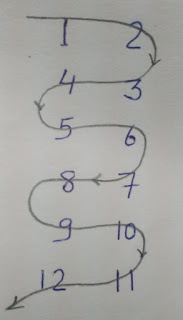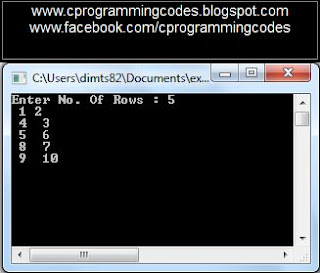## 3.27.2017

### Spiral Continuous two digit number pattern C Program

Q. Write a C program to make a Spiral tow digit number pattern as :

1 2
4 3
5 6
8 7
9 10
12 11

Ans.Figure: Spiral Continuous two digit number pattern C Program

/*spiral continuous tow digit number pattern C program source code*/
#include<stdio.h>
int main()
{
int num,r,c,p,q,x=1;
printf("Enter No. Of Rows : ");
scanf("%d", &num);

printf(" 1 2\n");
for(r=1; r<num ;r++)
{
if(r%2==0)
{
p=x+2;
for(c=1; c<=2; c++,x++,p++)
printf(" %d ",p);
}
else
{
q=x+3;
for(c=1; c<=2; c++,x++,q--)
printf(" %d ",q);
}
printf("\n");
}
getch();
return 0;
}

/************************
The output of above spiral continuous tow digit number pattern program would be:
*************************/Screen shot : Spiral Continuous two digit number pattern C Program Output

Related Top C Programs listing:

1.2.